UPSC  >  Exercise 7.4 and 7.5 NCERT Solutions - Triangles

# Exercise 7.4 and 7.5 NCERT Solutions - Triangles - NCERT Textbooks (Class 6 to Class 12) - UPSC

### Exercise 7.4

Q.1. Show that in a right-angled triangle, the hypotenuse is the longest side.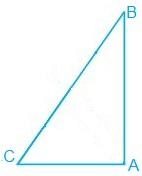Solution:
It is known that ABC is a triangle right angled at B.
We know that,
A + B + C = 180°
Now, if B + C = 90° then A has to be 90°.
Since A is the largest angle of the triangle, the side opposite to it must be the largest.
So, AB is the hypotenuse which will be the largest side of the above right-angled triangle i.e. ΔABC.

Q.2. In Fig. 7.48, sides AB and AC of ΔABC are extended to points P and Q, respectively. Also, PBC < QCB. Show that AC > AB.Solution:
It is given that PBC < QCB
We know that ABC + PBC = 180°
So, ABC = 180°-PBC
Also,
ACB + QCB = 180°
Therefore ACB = 180° -QCB
Now, since PBC < QCB,
∴ ABC > ACB
Hence, AC > AB as sides opposite to the larger angle is always larger.

Q.3. In Fig. 7.49, B < A and C < D. Show that AD < BC.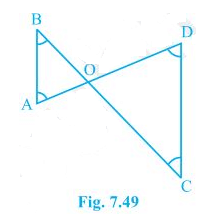Solution:
In the question, it is mentioned that angles B and angle C is smaller than angles A and D, respectively i.e. B < A and C < D.
Now,
Since the side opposite to the smaller angle is always smaller
AO < BO — (i)
And OD < OC —(ii)
By adding equation (i) and equation (ii) we get
AO + OD < BO + OC

Q.4. AB and CD are respectively the smallest and longest sides of a quadrilateral ABCD (see Fig. 7.50). Show that A > C and B > D.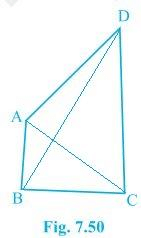Solution:
In ΔABD, we see that
So, ADB < ABD — (i) (Since angle opposite to longer side is always larger)
Now, in ΔBCD,
BC < DC < BD
Hence, it can be concluded that
BDC < CBD — (ii)
Now, by adding equation (i) and equation (ii) we get,
ADB + BDC < ABD + CBD
B > D
Similarly, In triangle ABC,
ACB < BAC — (iii) (Since the angle opposite to the longer side is always larger)
DCA < DAC — (iv)
By adding equation (iii) and equation (iv) we get,
ACB + DCA < BAC+DAC
∴ A > C

Q.5. In Fig 7.51, PR > PQ and PS bisect QPR. Prove that PSR > PSQ.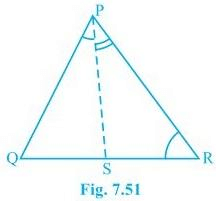Solution:
It is given that PR > PQ and PS bisects QPR
Now we will have to prove that angle PSR is smaller than PSQ i.e. PSR > PSQ
Proof:
QPS = RPS — (ii) (As PS bisects ∠QPR)
PQR > PRQ — (i) (Since PR > PQ as angle opposite to the larger side is always larger)
PSR = PQR + QPS — (iii) (Since the exterior angle of a triangle equals to the sum of opposite interior angles)
PSQ = PRQ + RPS — (iv) (As the exterior angle of a triangle equals to the sum of opposite interior angles)
PQR + QPS > PRQ + RPS
Thus, from (i), (ii), (iii) and (iv), we get
PSR > PSQ

Q.6. Show that of all line segments drawn from a given point not on it, the perpendicular line segment is the shortest.
Solution:
First, let “l” be a line segment and “B” be a point lying on it. A line AB perpendicular to l is now drawn. Also, let C be any other point on l. The diagram will be as follows: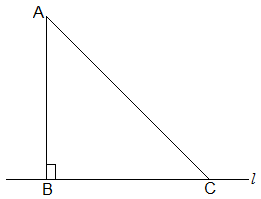To prove:
AB < AC
Proof:
In ΔABC, B = 90°
Now, we know that
A + B + C = 180°
∴ A + C = 90°
Hence, C must be an acute angle which implies C < B
So, AB < AC (As the side opposite to the larger angle is always larger)

### Exercise 7.5

Q.1. ABC is a triangle. Locate a point in the interior of ΔABC which is equidistant from all the vertices of ΔABC.
Soultion:
Let us consider a ΔABC.
Draw ‘l’ the perpendicular bisector of AB.
Draw ‘m’ the perpendicular bisector of BC.
Let the two perpendicular bisectors ‘l’ and ‘m’ meet at O. ‘O’ is the required point which is equidistant from A, B and C.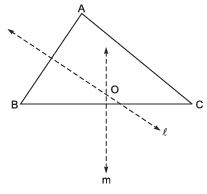Note: If we draw a circle with centre ‘O’ and radius OB or OC, then it will pass through A, B and C.

Q.2. In a triangle, locate a point in its interior which is equidistant from all the sides of the triangle.
Solution:
Let us consider a ΔABC.
Draw ‘l’ the bisector of ∠B.
Draw ‘m’ the bisector of ∠C.
Let the two bisectors l and m meet at O. Thus, ‘O’ is the required point which is equidistant from the sides of ΔABC.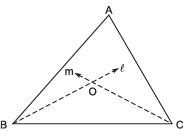required point which is equidistant from the sides of ΔABC.
Note: If we draw OM ⊥ BC and draw a circle with O as centre and OM as radius, then the circle will touch the sides of the triangle.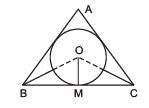Q.3. In a huge park, people are concentrated at three points (see figure): A : where there are different slides and swings form children,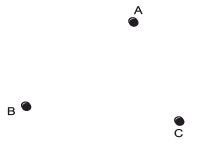B : near which a man-made lake is situated, C: which is near to a large parking and exit.
Where should an ice cream parlour be set up so that maximum number of persons can approach it?
Hint: The parlour should be equidistant from A, B and C.

Solution: Let us join A and B, and draw ‘l’ the perpendicular bisector of AB.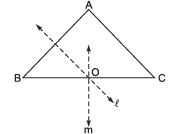Now, join B and C, and draw ‘m’ the perpendicular bisector of BC. Let the perpendicular bisectors ‘l’ and ‘m’ meet at ‘O’. The point ‘O’ is the required point where the ice cream parlour be set up.
Note: If we join ‘A’ and ‘C’, and draw the perpendicular bisectors, then it will also meet (or pass through) the point O.

Q.4. Complete the hexagonal and star shaped Rangolies [see Fig. (i) and (ii)] by filling them with as many equilateral triangles of side 1 cm as you can. Count the number of triangles in each case. Which has more triangles?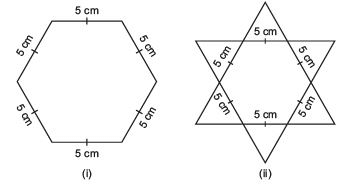Solution: It is an activity. We get the 150 equilateral triangles in the figure

(i) and 300 equilateral triangles in the figure

(ii). ∴ The figure

(ii) has more triangles.

The document Exercise 7.4 and 7.5 NCERT Solutions - Triangles | NCERT Textbooks (Class 6 to Class 12) - UPSC is a part of the UPSC Course NCERT Textbooks (Class 6 to Class 12).
All you need of UPSC at this link: UPSC

## FAQs on Exercise 7.4 and 7.5 NCERT Solutions - Triangles - NCERT Textbooks (Class 6 to Class 12) - UPSC

 1. What are the properties of an isosceles triangle?Ans. An isosceles triangle has two sides of equal length and two angles of equal measure. The base angles, formed by the equal sides, are always congruent.
 2. How can I determine if a triangle is equilateral?Ans. A triangle is equilateral if all three of its sides are equal in length. To determine if a triangle is equilateral, you can measure the lengths of its sides or check if all three angles are congruent.
 3. What is the Pythagorean Theorem?Ans. The Pythagorean Theorem states that in a right triangle, the square of the length of the hypotenuse (the side opposite the right angle) is equal to the sum of the squares of the other two sides. It is represented by the equation a² + b² = c², where c is the hypotenuse and a and b are the other two sides.
 4. How can I find the area of a triangle?Ans. The area of a triangle can be found using the formula A = (base × height) / 2. The base is the length of one of the sides, and the height is the perpendicular distance from the base to the opposite vertex.
 5. Can a triangle have more than one obtuse angle?Ans. No, a triangle cannot have more than one obtuse angle. By definition, an obtuse angle measures greater than 90 degrees. Since the sum of the angles in a triangle is always 180 degrees, having more than one obtuse angle would result in a total angle measure greater than 180 degrees, which is not possible.

## NCERT Textbooks (Class 6 to Class 12)

1404 docs|679 tests

## NCERT Textbooks (Class 6 to Class 12)

1404 docs|679 tests

### How to Prepare for UPSC

Read our guide to prepare for UPSC which is created by Toppers & the best Teachers
Signup to see your scores go up within 7 days! Learn & Practice with 1000+ FREE Notes, Videos & Tests.
10M+ students study on EduRev
Track your progress, build streaks, highlight & save important lessons and more!(Scan QR code)
Related Searches

,

,

,

,

,

,

,

,

,

,

,

,

,

,

,

,

,

,

,

,

,

;